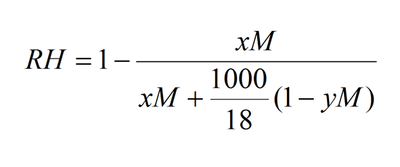The Relative Humidity (RH) in equilibrium with solutions can be understood in terms of Raoult's law.  It has two aspects that are counter-intuitive and lead to some surprising observations. The first is that the number of equivalent molecules in solution must be accounted for. This means that for sodium chloride, each ion in solution counts as a molecular equivalent. This requires knowledge of the ionization behaviour of the substance in solution. For example, ammonium sulfate effectively dissociates into two ions [NH4+ and (NH4SO4)-] and not three as might be expected.  Raoult's law starts to break down for PEG solutions over a molecular weight of 1000 Da but this can be corrected using the Flory-Huggins model for the entropy of mixing (used in equation 2).The parameter y can be calculated from this equation: y= (1/ρ)m/1000, where ρ is the density of the salt and m is the molecular weight.